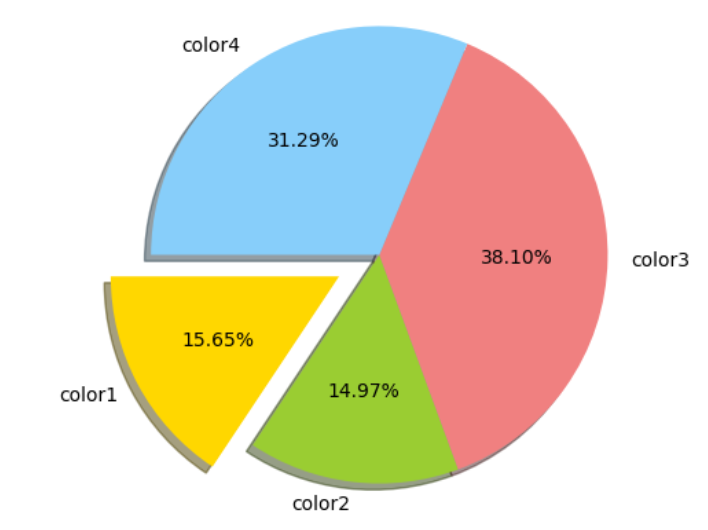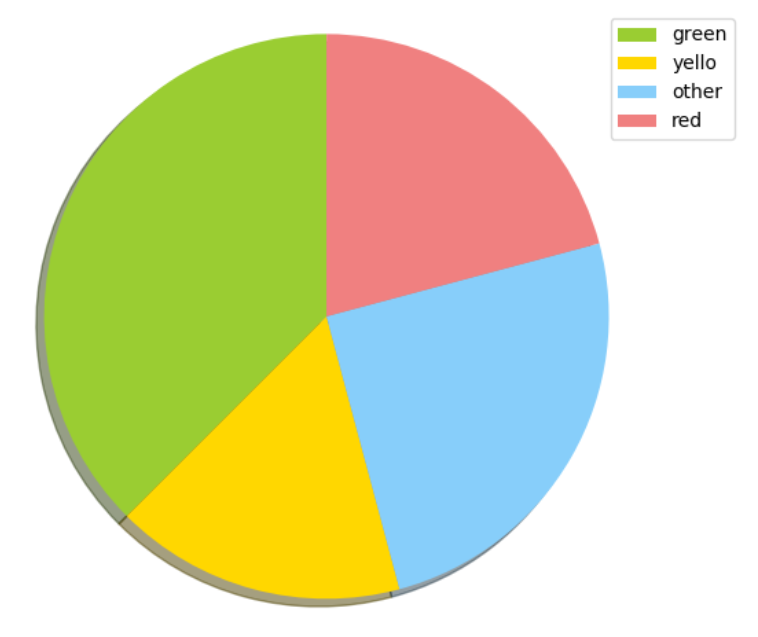Search

# Data Visualization In Machine Learning | Machine Learning Project Help

Data visualization is the representation of data or information in a graph, chart, or other visual formats. It communicates the relationships of the data with images.

Data visualization is used in a large number of areas in statistics and machine learning.

There are five key plots that you need to know well for basic data visualization. They are:

• Line Plot

• Bar Chart

• Histogram Plot

• Box and Whisker Plot

• Pie chart

• Scatter chart

• Series chart

• Mosaic chart

• Heat Map

### Line Plot

A line plot is generally used to present observations collected at regular intervals.

The x-axis represents the regular interval, such as time. The y-axis shows the observations, ordered by the x-axis and connected by a line.

A line plot can be created by calling the plot() function and passing the x-axis data for the regular interval, and y-axis for the observations.

Line plot is a type of chart that displays information as a series of data points connected by straight line segments.

Line plots are generally used to visualize the directional movement of one or more data over time. In this case, the X axis would be DateTime and the y axis contains the measured quantity, like, stock price, weather, monthly sales, etc.

```# create line plot
pyplot.plot(x, y)```### Bar Chart

A bar chart or bar graph is a chart or graph that presents categorical data with rectangular bars with heights or lengths proportional to the values that they represent. The bars can be plotted vertically or horizontally.

Draw vertically:

Example:

```
import matplotlib.pyplot as plt; plt.rcdefaults()
import numpy as np
import matplotlib.pyplot as plt

objects = ('red', 'green', 'yellow', 'blue', 'orange', 'pink')
y_pos = np.arange(len(objects))
performance = [15,12,10,5,4,1]

plt.barh(y_pos, performance, align='center', alpha=0.5)
plt.xticks(y_pos, objects)
plt.ylabel('Value')
plt.title('Color usage')

plt.show()```

Output:Draw horizontally:

To draw horizontally used the function barh()

Example:

```import matplotlib.pyplot as plt; plt.rcdefaults()
import numpy as np
import matplotlib.pyplot as plt

objects = ('red', 'green', 'yellow', 'blue', 'orange', 'pink')
y_pos = np.arange(len(objects))
performance = [15,12,10,5,4,1]

plt.barh(y_pos, performance, align='center', alpha=0.5)
plt.xticks(y_pos, objects)
plt.ylabel('Value')
plt.title('Color usage')

plt.show()```

Output:### Histogram plot:

A histogram shows the frequency on the vertical axis and the horizontal axis is another dimension. Usually, it has bins, where every bin has a minimum and maximum value. Each bin also has a frequency between x and infinite.

Example:

```import numpy as np
import matplotlib.mlab as mlab
import matplotlib.pyplot as plt

x = [15,18,17,2,4,3,55,8,9,40,61,12,33,22,35,36,36,14,46,45]
num_bins = 5
n, bins, patches = plt.hist(x, num_bins, facecolor='blue', alpha=0.5)
plt.show()```

Output:### Box and Whisker Plot

A box plot which is also known as a whisker plot displays a summary of a set of data containing the minimum, first quartile, median, third quartile, and maximum.Drawing a Box Plot

Boxplot can be drawn calling Series.box.plot() and DataFrame.box.plot(), or DataFrame.boxplot() to visualize the distribution of values within each column.

Example

```import pandas as pd
import numpy as np
df = pd.DataFrame(np.random.rand(15, 5), columns=['Box1', 'Box2', 'Box3', 'Box4', 'Box5'])
df.plot.box(grid='True')```

Output:### Pie Chart

Matplotlib pie chart

First import matplotlib as:

`import matplotlib.pyplot as plt`

Example:

```
import matplotlib.pyplot as plt
# Data to plot
labels = 'color1', 'color2', 'color3', 'color4'
sizes = [115, 110, 280, 230]
colors = ['gold', 'yellowgreen', 'lightcoral', 'lightskyblue']
explode = (0.2, 0, 0, 0) # explode 1st slice
# Plot
plt.pie(sizes, explode=explode, labels=labels, colors=colors,
plt.axis('equal')
plt.show()```

Output:With “Legend”

```import matplotlib.pyplot as plt
labels = ['green', 'yello', 'other', 'red']
sizes = [45, 20, 30, 25]
colors = ['yellowgreen', 'gold', 'lightskyblue', 'lightcoral']
patches, texts = plt.pie(sizes, colors=colors, shadow=True, startangle=90)
plt.legend(patche
s, labels, loc="best")
plt.axis('equal')
plt.tight_layout()
plt.show()```

Output:### Scatter Plot

Use the scatter() method to draw a scatter plot diagram:

Example:

```import matplotlib.pyplot as plt
x = [2,1,10,8,5,15]
y = [45,54,56,55,110,78]
plt.scatter(x, y)
plt.show()```

Output:### Series chart

There are many ways to draw the time-series graph:

• Line Plots.

• Histograms and Density Plots.

• Box and Whisker Plots.

• Heat Maps.

• Lag Plots or Scatter Plots.

• Autocorrelation Plots.

### Mosaic chart

These charts are a good representation of categorical entries. A mosaic plot allows visualizing multivariate categorical data in a rigorous and informative way.

Example

```from statsmodels.graphics.mosaicplot import mosaic
import matplotlib.pyplot as plt
import pandas

gender = ['male', 'male', 'male', 'female', 'female', 'female']
pet = ['cat', 'dog', 'dog', 'cat', 'dog', 'cat']
data = pandas.DataFrame({'gender': gender, 'pet': pet})
mosaic(data, ['pet', 'gender'])
plt.show()
```

Output:### Heat Map

It shows the 2D representation of data.

Example:

```import numpy as np
import matplotlib.pyplot as plt
data = np.random.random((8, 8))
plt.imshow(data, cmap='cool', interpolation='nearest')
plt.show()```

imshow(), function use to draw the heat map

Output:Contact us for this machine learning assignment Solutions by Codersarts Specialist who can help you mentor and guide for such machine learning assignments.

If you have project or assignment files, You can send at contact@codersarts.com directly